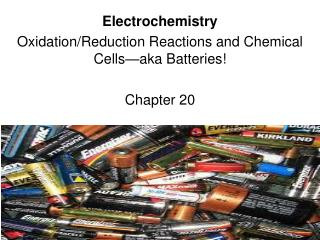DownloadDownload PresentationElectrochemistry Oxidation/Reduction Reactions and Chemical Cellsâ€”aka Batteries! Chapter 20

# Electrochemistry Oxidation/Reduction Reactions and Chemical Cellsâ€”aka Batteries! Chapter 20

Download Presentation## Electrochemistry Oxidation/Reduction Reactions and Chemical Cellsâ€”aka Batteries! Chapter 20

- - - - - - - - - - - - - - - - - - - - - - - - - - - E N D - - - - - - - - - - - - - - - - - - - - - - - - - - -
##### Presentation Transcript

1. Electrochemistry Oxidation/Reduction Reactions and Chemical Cells—aka Batteries! Chapter 20

2. Electrochemistry • Study of the relationship between chemical reactions and electricity. • Examples: • Batteries • Decomposing water • Rusting • Respiration • Electroplating

3. What’s involved in electrochemistry? • Obviously… • A Chemical Reaction and Electricity • Where does the electricity come from? • Moving Electrons

4. Moving Electrons? • We have to keep track of the electrons. • Reduction: gaining electrons • Oxidation: losing electrons • Hence, RedOx!

5. So how do we keep track of electrons? • You have to figure out how many electrons EACH ELEMENT has in a chemical formula AND • How many electrons EACH ELEMENT apparently has in a chemical bond. • This is called the “Oxidation Number”

6. Rules for AssigningOxidation Numbers Remember that in a chemical bond electrons are shared and givenor taken. This all has to do with electronegativity. Here are the rules:

7. Oxidation Number Rules ex: O2(g) and Fe(s) 2. The oxidation number of a monatomic ion is the same as the charge of the ion. (see your common ion sheet for others) ex: NaCl (Na = +1, Cl = -1). Elements in their elemental, uncombined state have an oxidation number of zero. 3. Hydrogen will have an oxidation number of +1. ex: HCl (H = +1, Cl = -1) Exception: in metal hydrides, hydrogen has an oxidation number of -1. ex: NaH (Na = +1, H = -1)

8. Oxygen has an oxidation number of -2. Oxidation Number Rules ex: H2O (H = +1, O=-2) Exception: in peroxides, oxygen has an oxidation number of -1 (because there are 2 O) ex: CaO2 (Ca = +2, O = -1) Exception: in OF2 oxygen has an oxidation number of +2 ex: CaCl2 (Ca=+2, Cl=-1x2, total=0) • Oxidation numbers must follow conservation of charge • in other words, the charges of the individual atoms must add up to the overall charge of the molecule or ion.

9. More Examples Cu(s) Cu=0 Ag= +1; N=+5; O=-2x3=-6 AgNO3(aq) I2(g) I=0 Sn=+2; Cl=-1x2=-2 SnCl2(s) NO3-1(aq) N=+5; O=-2x3=-6 SO4-2(aq) S=+6; O=-2x4=-8 Cd(OH)2(s) Cd=+2; (OH)=-1x2=-2 NiO2(s) Ni=+4; O=-2x2=-4

10. Oil Rig Oxidation Is Loss Reduction Is Gain Of electrons

11. Example: 2HCl + Mg  MgCl2 + H2 Mg = 0 H = +1 Cl = -1 H = 0 Cl = -1 Mg = +2 Identify how the elements’ charges are changing: Hydrogen: +1  0 Oxidation number is reduced H is reduced Magnesium: 0  +2 Oxidation number is increased Mg is oxidized What is H being reduced by? Mg  Mg is the “reducing agent” What is Mg being oxidized by? H  H is the “oxidizing agent” Now let’s write the half reactions

12. Example: 2HCl + Mg  MgCl2 + H2 Mg = 0 H = +1 Cl = -1 H = 0 Cl = -1 Mg = +2 Hydrogen is reduced, so it will be the reduction half reaction: Magnesium is oxidized, so it will be the oxidation half reaction: 2H+1 + 2e- H2 Mg  Mg+2 + 2e-

13. Another Example Sn+2 + 2Fe+3 Sn+4 + 2Fe+2 Sn = +2 Sn = +4 Fe = +2 Fe = +3 Iron is reduced, so it will be the reduction half reaction: Tin is oxidized, so it will be the oxidation half reaction: 2Fe+3 + 2e- 2Fe+2 Sn+2  Sn+4 + 2e-

14. Book Problems p. 884 # 20.11, 20.12, # 20.15ab, 20.16ab